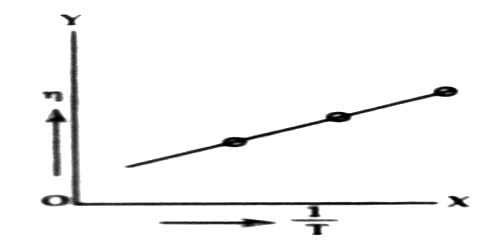# Effect of Temperature on Co-efficient of Viscosity in Liquids

Effect of temperature on co-efficient of viscosity in Liquids

Co-efficient of viscosity is affected by temperature. Experimental results show that with the increase of temperature co-efficient of viscosity decreases. For example, the co-efficient of viscosity of water at 80°C is one third the co-efficient of viscosity at 0°C.

Explanation with the help of molecular theory:

We know, a tangential dragging force acts between two adjacent layers of a fluid in motion. This force is called viscous force. Intermoleculer force among the molecules of two layers is the cause of this viscous force. Viscous force is dependent on the interatomic distance. When temperature increases interatomic distance also increases. The impact of increasing the temperature of a liquid is to reduce the cohesive forces while simultaneously increasing the rate of molecular interchange.As a result the interactomic force decreases consequently viscous force decreases (figure). According to equation (1) co-efficient of viscosity also decreases. The relation between temperature and co-efficients of viscosity follows the relation:

log η = A + B/T

where A and B are constant and T is the temperature in kelvin scale. Now if log η versus 1/T graph is plotted, it will be a straight line.